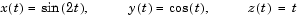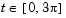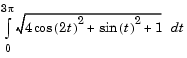MathematicsExample: Computing the Length of a Curve

You can use `quad` or `quadl` to compute the length of a curve. Consider the curve parameterized by the equations

•where.

A three-dimensional plot of this curve is

• ```t = 0:0.1:3*pi;
plot3(sin(2*t),cos(t),t)
```

The arc length formula says the length of the curve is the integral of the norm of the derivatives of the parameterized equations

•The function `hcurve` computes the integrand

• ```function f = hcurve(t)
f = sqrt(4*cos(2*t).^2 + sin(t).^2 + 1);
```

Integrate this function with a call to `quad`

• ```len = quad(@hcurve,0,3*pi)

len =
1.7222e+01
```

The length of this curve is about 17.2.Numerical Integration (Quadrature) Example: Double Integration© 1994-2005 The MathWorks, Inc.